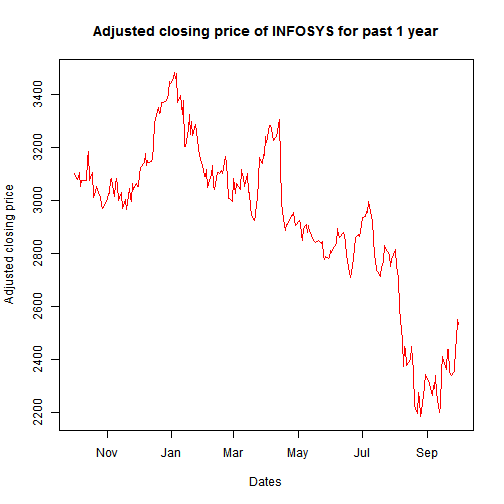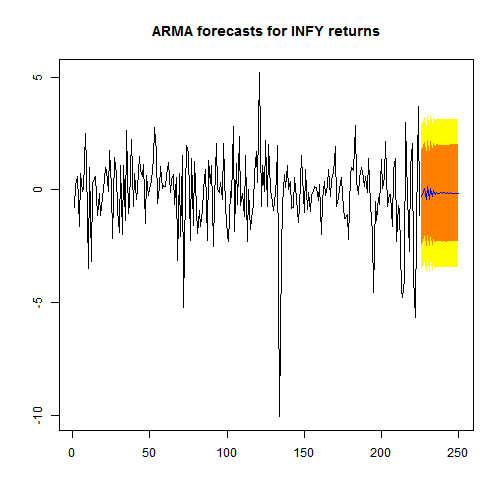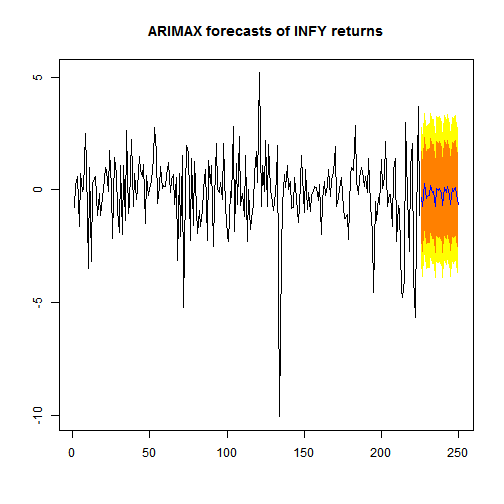Want to share your content on R-bloggers? click here if you have a blog, or here if you don't.

Why is it important?

# Why is it important?

India has a lot to achieve in terms of becoming a developed nation from an economic standpoint. An aspect which, in my opinion, is of utmost importance is the formation of structurally sound and robust financial markets. A prerequisite for that is active participation of educated and informed traders in the market place which would result in better price discovery and in turn better functioning market in general.

Statistical modelling techniques supplemented with some subject understanding could be an informed trading strategy. In the long run it might not be possible to outplay the market using a simple backward looking statistical model, but in the short run intelligent estimates based on model and subject matter expertise could prove to be helpful. In our previous posts with Infosys stock prices, we used basic visualization and simple linear regression techniques to try and predict the future returns from historical returns. Lets step on the pedal and move over to some more sophisticated techniques to do the same. We will start with the same basics of running basic checks on the data and then take a deeper dive in terms of modelling technique to use.

data <- read.csv("01-10-2010-TO-01-10-2011INFYEQN.csv")summary(data)
##         Date      Close.Price  ##  01-Apr-11:  1   Min.   :2183  ##  01-Aug-11:  1   1st Qu.:2801  ##  01-Dec-10:  1   Median :2993  ##  01-Feb-11:  1   Mean   :2929  ##  01-Jul-11:  1   3rd Qu.:3106  ##  01-Jun-11:  1   Max.   :3481  ##  (Other)  :245
plot(as.Date(data$Date, "%d-%b-%y"), data$Close.Price, xlab = "Dates", ylab = "Adjusted closing price",     type = "l", col = "red", main = "Adjusted closing price of INFOSYS for past 1 year")There seems to be a lot of randomness in the series and the adf.test results prove that the series is non-stationary (I(1)). Which means that the series will have to be first differenced to make is stationary. (Refer to this post for more understanding on stationarity).

library(tseries, quietly = T)
adf.test(data$Close.Price) ## ## Augmented Dickey-Fuller Test## ## data: data$Close.Price ## Dickey-Fuller = -2.451, Lag order = 6, p-value = 0.3858## alternative hypothesis: stationary
infy_ret <- 100 * diff(log(data$Close.Price)) # Auto-regressive moving average (ARMA) model There is one primary difference between time series and cross sectional datasets and that is the presence of auto-correlation in time series data. The concept of auto-correlation is not applicable to cross sectional regression as there is no dependence in the observations. However, there is explicit dependent of time series' future value on its near past values. We arrive at the estimates in a time series model after solving the Yule Walker equations unlike MLE or simple OLS techniques in the case of cross sectional linear regressions. The idea of an ARMA model is fairly intuitive to understand, however, the math gets extremely tricky. We will take a crack at explaining what an ARMA model is in laymen language. A typical ARMA(1,1) model can be expressed as : $\begin{equation} z_t = \alpha + \phi z_{t-1} + \theta\epsilon_{t-1} + \epsilon_t \end{equation}$ The (1,1) in the equation stand for the auto-regressive($$z_{t}$$) and moving average($$\epsilon_{t}$$) lag orders respectively. The intuitive understanding of the above equation is pretty straightforward. The current value of the time series $$z_t$$ will depend on the past value of the series $$z_{t-1}$$ and will correct itself to the error made in the last time period $$\epsilon_{t-1}$$. Lets try and fit an ARMA model to our INFY returns data and see how the results turn out. summary(arma(infy_ret, order = c(2, 2))) ## ## Call:## arma(x = infy_ret, order = c(2, 2))## ## Model:## ARMA(2,2)## ## Residuals:## Min 1Q Median 3Q Max ## -9.5723 -0.9214 -0.0719 0.9289 4.5550 ## ## Coefficient(s):## Estimate Std. Error t value Pr(>|t|) ## ar1 -0.34007 0.02591 -13.12 <2e-16 ***## ar2 -0.97524 0.01817 -53.67 <2e-16 ***## ma1 0.44427 0.00702 63.31 <2e-16 ***## ma2 1.01522 0.00802 126.60 <2e-16 ***## intercept 0.00525 0.21054 0.02 0.98 ## ---## Signif. codes: 0 '***' 0.001 '**' 0.01 '*' 0.05 '.' 0.1 ' ' 1 ## ## Fit:## sigma^2 estimated as 2.9, Conditional Sum-of-Squares = 718.3, AIC = 985.8 We can see that AR as well as MA coefficients are all significant at 99%, evident from the small p-values. Since our objective here is to forecast future returns lets evaluate the performance of the ARMA model in terms of out-of-sample forecast performance. For this we will divide the data into 2 parts, on one we will train the model and on the other we will test the out-of-sample forecast ability. Here Wehave used ARIMA function to fit the model as the object type “arima” is easily compatible with forecast() and predict() function. ARIMA is nothing by a normal ARMA model with the order of integration included as an argument to the function. In our case, our series was I(1) but we have first differenced it already so in the ARIMA function we will keep the “I” part = 0. library(forecast, quietly = T) infy_ret_train <- infy_ret[1:(0.9 * length(infy_ret))] # Train datasetinfy_ret_test <- infy_ret[(0.9 * length(infy_ret) + 1):length(infy_ret)] # Test datasetfit <- arima(infy_ret_train, order = c(2, 0, 2))arma.preds <- predict(fit, n.ahead = (length(infy_ret) - (0.9 * length(infy_ret))))$predarma.forecast <- forecast(fit, h = 25)plot(arma.forecast, main = "ARMA forecasts for INFY returns")accuracy(arma.preds, infy_ret_test)  # RMSE values
##  RMSE ## 2.489

Above are the results that we obtain with a simple ARMA(2,2) model. The orange and yellow region provide us the 99% and 95% confidence level for the forecasts respectively. An intrinsic shortcoming of ARMA models, which is evident from the plot above, is the assumption of mean reversion of the series. What this means is that after some time in future the forecasts would tend to the mean of the time series $$z_{t}$$'s historical values thus making it a poor model for long term predictions.

Now, there are some intuitive variables that one can introduce in the model based on subjective understanding to improve the model. In cases where one wishes to augment a simple univariate time series regression with some exogenous set of variable, ARIMAX function can be employed. In cases where the additional variables could have a feedback relation with the time series in question (i.e they are endogenous) one can employ Vector auto regressive (VAR) models. Let me try and elaborate a little on them before I start to sound confusing. In our example above in question, lets say that our hypothesis is that day of the week has an effect on the stock prices. To include this in our model all that we need is 4 new dummy variables for 4 days of the week (5th one by default goes to the intercept) and include them in the above ARMA model using ARIMAX function. Here these dummy variables will be completely exogenous to our dependent variable (INFY returns), because no matter how/what the stock price is for INFY, its not going to affect the day of the week! However, lets say we wanted to include NIFTY returns as an additional variable in the analysis, a VAR model would be preferable. The reason being that there could be a feedback relation between INFY returns and NIFTY returns which might be ignored if we use a simple ARIMAX function.

# ARIMA model with day of the week variable

We will try and illustrate with an example the former where we will use day of the week as an exogenous variable to augment our ARMA model for INFY returns. The ARIMAX model can be simply written as:

$\begin{equation} z_t = \alpha + \phi z_{t-1} + \theta\epsilon_{t-1} + \gamma x_t + \epsilon_t \end{equation}$

where, $$x_{t}$$ is the exogenous variable. In our case we will have 4 dummy variables created for the 4 days.

data$day <- as.factor(weekdays(as.Date(data$Date, "%d-%b-%y")))days <- data$day[2:nrow(data)]xreg1 <- model.matrix(~as.factor(days))[, 2:5]colnames(xreg1) <- c("Monday", "Thursday", "Tuesday", "Wednesday")fit2 <- arima(infy_ret_train, order = c(2, 0, 2), xreg = xreg1[c 1="*" 2="length(infy_ret)))," 3="fit1.preds <- forecast(fit2, h = 25, xreg = xreg1[c language="(226:250),"][/c])fit1.preds <- predict(fit2, n.ahead = 25, newxreg = xreg1[c language="(226:250),"][/c])plot(forecast(fit2, h = 20, xreg = xreg1[c language="(226:250),"][/c]), main = "ARIMAX forecasts of INFY returns")accuracy(fit1.preds$pred, infy_ret_test)
##  RMSE ## 2.431

The performance of the ARIMA model with weekdays factor variable seems to be better than a simple ARMA model which is evident from the lower RMSE of the ARIMAX model. This is just one example of variables that could be used to augment a simple ARMA model, there could be many more variants of such variables that might further increase the performance of the model. In the next post we would try to cover vector auto regression and how/when it can be used.

Feedback/criticisms welcome.